1 2

# 基础作业一

6-1 求两数平方根之和 （10 分)

double fun (double a, double b);

#include<stdio.h>
#include <math.h>
double fun (double *a, double *b);
int main ( )
{ double a, b, y;
scanf ("%lf%lf", &a, &b );
y=fun(&a, &b); printf ("y=%.2f\n", y );
return 0;
}

/* 请在这里填写答案 */

12 20

y=7.94

## （一）实验代码：

double fun (double *a, double *b)
{
double y;
y=pow(*a,0.5)+pow(*b,0.5);
return y;
}

## （二）设计思路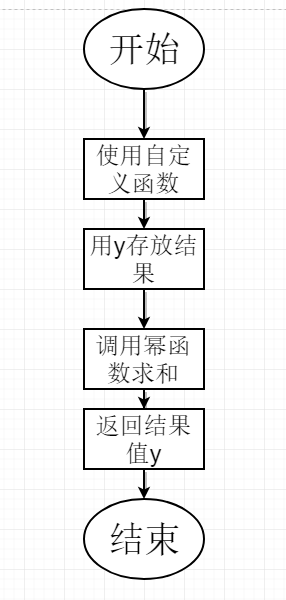## （四）运行结果截图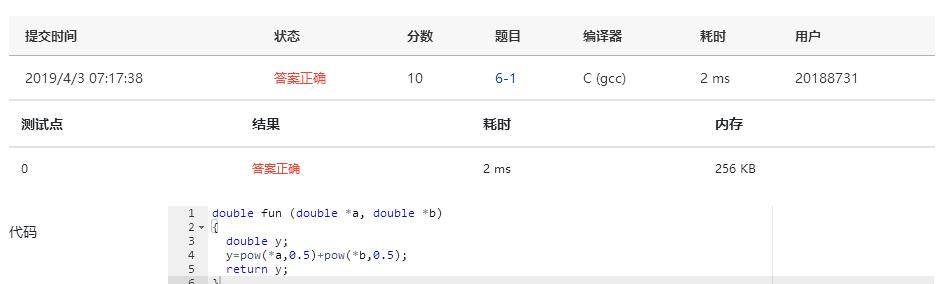# 基础题二

7-1 利用指针返回多个函数值 （30 分)

5
8 9 12 0 3

max = 12
min = 0

## （一）实验代码：

#include<stdio.h>
void max_min(int a[], int n, int *pmax, int *pmin);
int main()
{
int i,n,max,min;
scanf("%d",&n);
int a[n];
for(i=0;i<n;i++){
scanf("%d",&a[i]);
}
max_min(a,n,&max,&min);  //把max和min地址赋给指针变量
printf("max = %d\nmin = %d",max,min);

return 0;
}
void max_min(int a[],int n, int *pmax, int *pmin)
{
int max,min,k,i,t;
for(k=0;k<n-1;k++){   //选择排序
max=k;
for(i=k+1;i<n;i++)
if(a[i]>a[max])
max=i;
t=a[max];
a[max]=a[k];
a[k]=t;
}
*pmax=a; //第一个数最大
*pmin=a[n-1];  //倒数第二个数最小
}

## （二）设计思路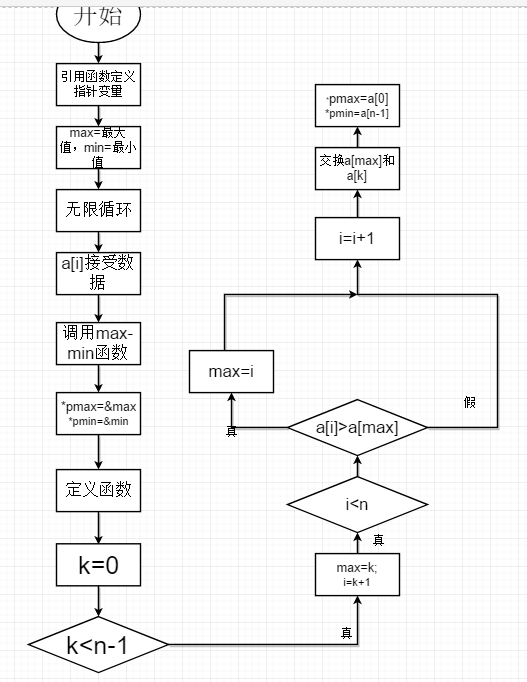## （三）实验遇到的问题及解决方案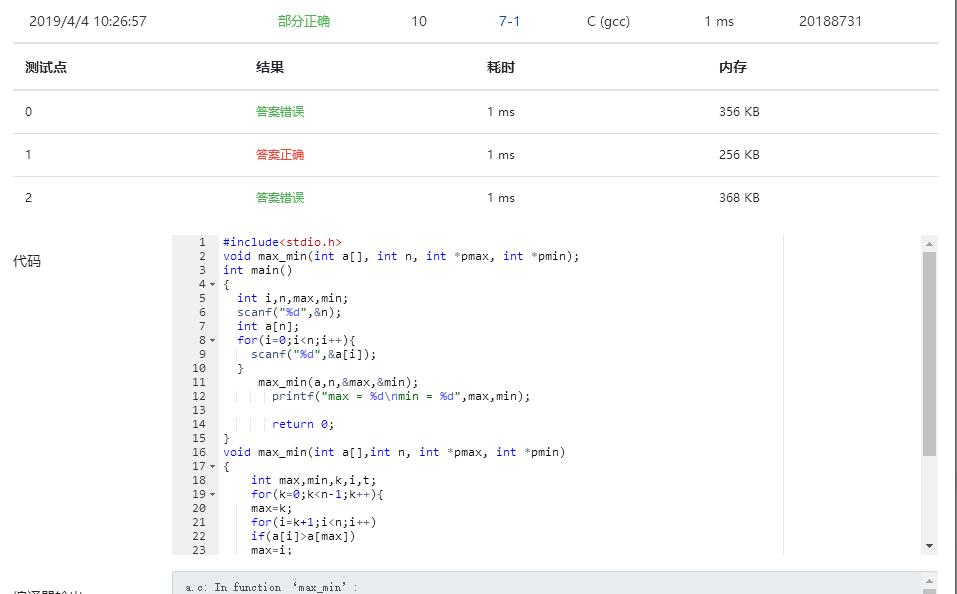## （四）运行结果截图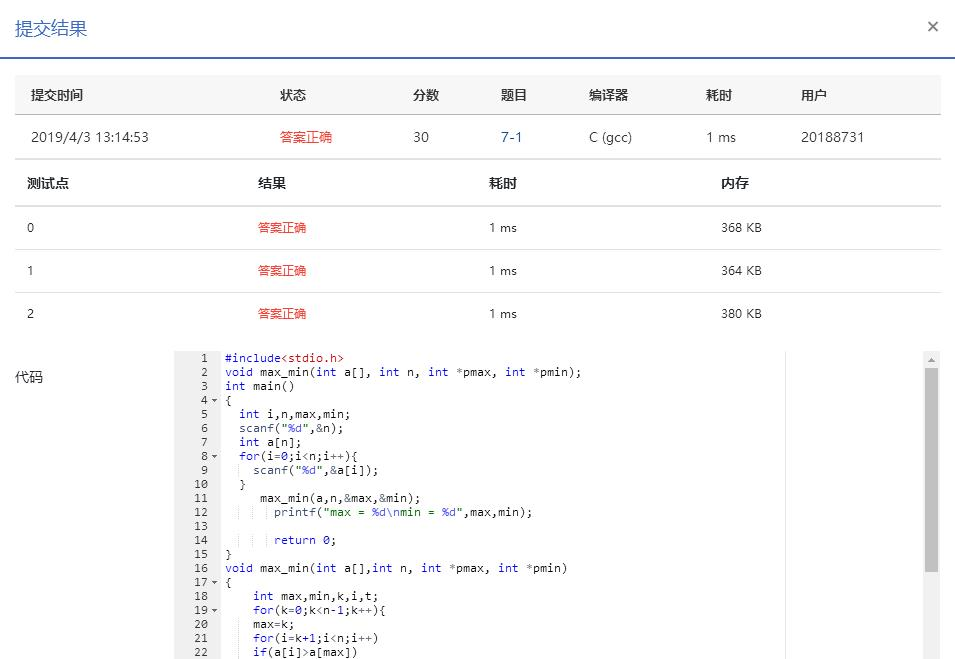## 思考题

### （一）为什么要使用指针？它有什么用？

1.处理堆中存放的大型数据.2用于.快速访问类的成员数据和函数.3.以别名的方式向函数传递参数。好处有1

## 预习题

6-3 最小数放前最大数放后 （10 分)

void input(int *arr,int n);
void max_min(int *arr,int n);
void output(int *arr,int n);

input函数的功能是输入 n个元素存到指针arr所指向的一维数组中。

max_min函数的功能是求指针arr所指向的一维数组中的最大值和最小值，其中最小的数与第一个数对换，将最大的数与最后一个数对换。

output函数的功能是在一行中输出数组元素，每个元素输出占3列。

#include<stdio.h>
void input(int *arr,int n);
void max_min(int *arr,int n);
void output(int *arr,int n);
int main()
{ int a;
input(a,10);
max_min(a,10);
output(a,10);
return 0;
}

/* 请在这里填写答案 */

5 1 4 8 2 3 9 5 12 7

  1  5  4  8  2  3  9  5  7 12

## （一）实验代码：

void input(int *arr,int n)
{
int i;
for(i=0;i<n;i++)
{
scanf("%d",&arr[i]);
}
}
void max_min(int *arr,int n)
{
int i,t, max,min,a=0,b=0;
min = max = arr;
for(i=0;i<10;i++)
{
if(max <= arr[i])
{
max = arr[i];
a = i;
}
if(min >= arr[i])
{
min = arr[i];
b = i;
}
}
t = arr;
arr = arr[a];
arr[a] = t;
t = arr;
arr = arr[b];
arr[b] = t;
}
void output(int *arr,int n)
{
int i;
for(i=0;i<n;i++)
{
printf("%3d",arr[i]);
}
}

## （二）设计思路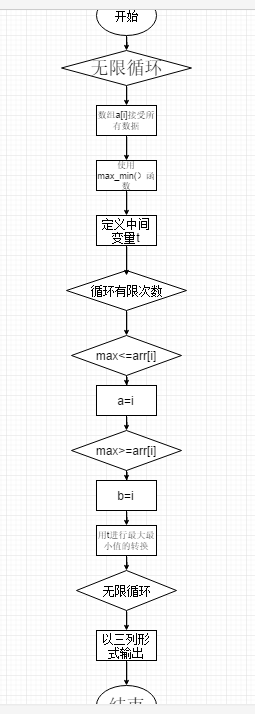## （三）实验遇到的问题及解决方案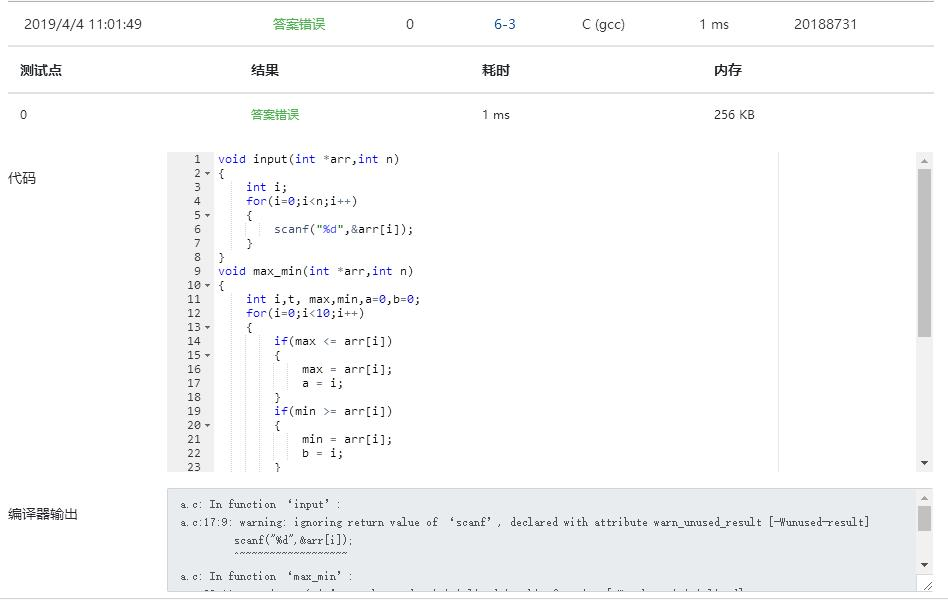## （四）运行结果截图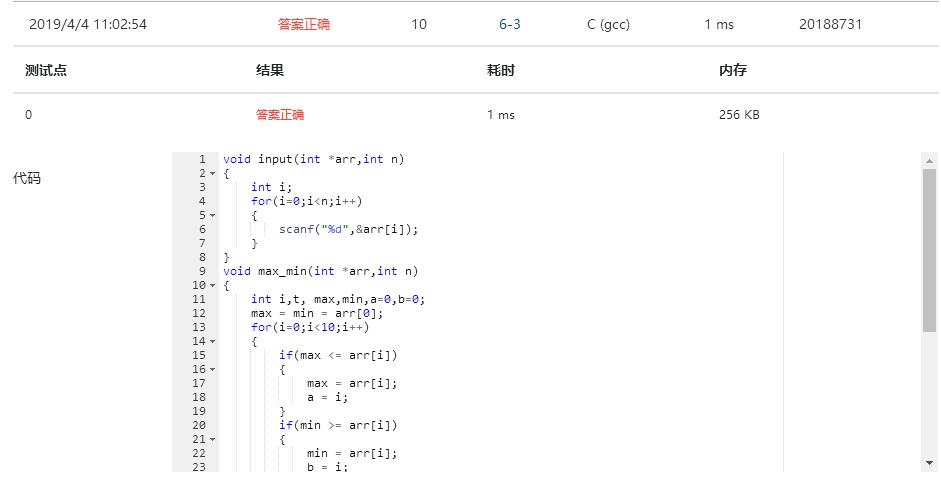## 学习进度

 周/日期 这周所花的时间 代码行 学到的知识点简介 目前比较迷惑的问题 3/31-4/5 2天 94 指针的定义及其基本类型 指针是否对任意函数都适用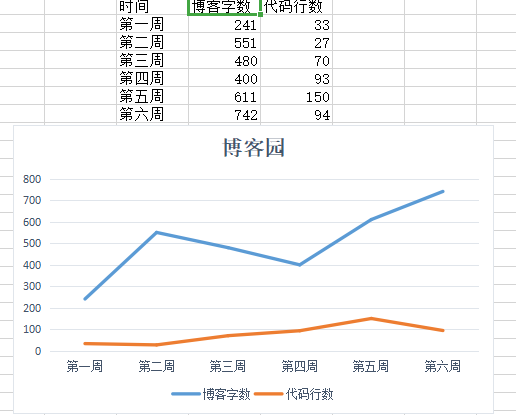posted @ 2019-04-04 17:54  小嗝嗝爱作业  阅读(...)  评论(... 编辑 收藏